Next: 3.1.1.3 Thermoelectric Figure of Merit Up: 3.1.1 Ballistic Thermoelectric Properties of AGNRs Previous: 3.1.1.1 Electronic Properties   Contents

### 3.1.1.2 Phononic Properties

The ballistic transmission function of phonons, which is the number of phononic modes at some energy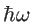(Eq. 2.42), is shown in Fig. 3.4. As expected, the transmission function increases with the ribbon's width. In addition, the phononic window function is shown in the inset of Fig. 3.4 at various temperatures. This function, which qualifies the contribution of different phonon frequencies in the thermal conductance, increases with temperature. Therefore, at higher temperatures high energy phonons will contribute to the thermal transport as well.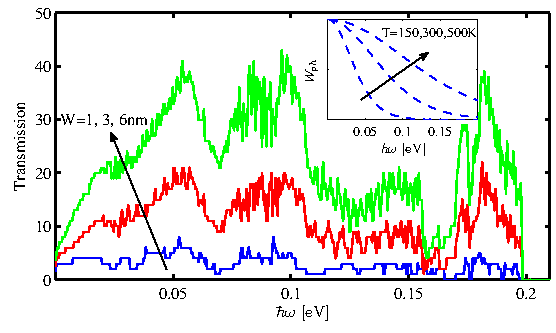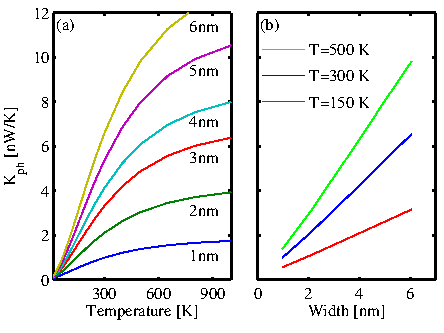Figure 3.5-a and -b show the ballistic thermal conductance of AGNRs as a function of temperature and the ribbon's width, respectively. By increasing the temperature and thus the width of the phononic window function, the thermal conductance increases. However, as the energy spectrum of AGNRs is limited to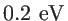(Fig. 3.4), the thermal conductance saturates at very high temperatures (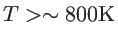). As shown in Fig. 3.5-b, the thermal conductance increases linearly with the ribbon's width. In fact, the ballistic thermal conductance divided by the width is constant. This is due to the fact that by increasing the width and thus the number of carbon atoms in the unit cell, the number of modes increases almost linearly in the whole energy spectrum, and, therefore, the ballistic transmission function is directly proportional to the width.Next: 3.1.1.3 Thermoelectric Figure of Merit Up: 3.1.1 Ballistic Thermoelectric Properties of AGNRs Previous: 3.1.1.1 Electronic Properties   Contents
H. Karamitaheri: Thermal and Thermoelectric Properties of Nanostructures